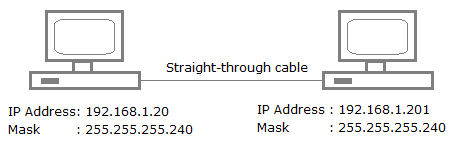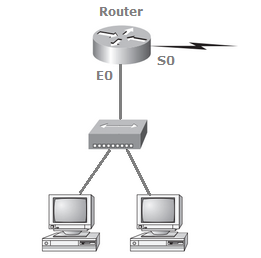Courses

# Subnetting, Networking Quiz

## 20 Questions MCQ Test Placement Papers - Technical & HR Questions | Subnetting, Networking Quiz

Description
This mock test of Subnetting, Networking Quiz for Quant helps you for every Quant entrance exam. This contains 20 Multiple Choice Questions for Quant Subnetting, Networking Quiz (mcq) to study with solutions a complete question bank. The solved questions answers in this Subnetting, Networking Quiz quiz give you a good mix of easy questions and tough questions. Quant students definitely take this Subnetting, Networking Quiz exercise for a better result in the exam. You can find other Subnetting, Networking Quiz extra questions, long questions & short questions for Quant on EduRev as well by searching above.
QUESTION: 1

### Your router has the following IP address on Ethernet0: 172.16.2.1/23. Which of the following can be valid host IDs on the LAN interface attached to the router? 172.16.1.100 172.16.1.198 172.16.2.255 172.16.3.0

Solution: The router's IP address on the E0 interface is 172.16.2.1/23, which is 255.255.254.0. This makes the third octet a block size of 2. The router's interface is in the 2.0 subnet, and the broadcast address is 3.255 because the next subnet is 4.0. The valid host range is 2.1 through 3.254. The router is using the first valid host address in the range.
QUESTION: 2

### Which two statements describe the IP address 10.16.3.65/23? The subnet address is 10.16.3.0 255.255.254.0. The lowest host address in the subnet is 10.16.2.1 255.255.254.0. The last valid host address in the subnet is 10.16.2.254 255.255.254.0. The broadcast address of the subnet is 10.16.3.255 255.255.254.0.

Solution: The mask 255.255.254.0 (/23) used with a Class A address means that there are 15 subnet bits and 9 host bits. The block size in the third octet is 2 (256 - 254). So this makes the subnets in the interesting octet 0, 2, 4, 6, etc., all the way to 254. The host 10.16.3.65 is in the 2.0 subnet. The next subnet is 4.0, so the broadcast address for the 2.0 subnet is 3.255. The valid host addresses are 2.1 through 3.254.
QUESTION: 3

### A network administrator is connecting hosts A and B directly through their Ethernet interfaces, as shown in the illustration. Ping attempts between the hosts are unsuccessful. What can be done to provide connectivity between the hosts?A crossover cable should be used in place of the straight-through cable. A rollover cable should be used in place of the straight-through cable. The subnet masks should be set to 255.255.255.192. A default gateway needs to be set on each host. The subnet masks should be set to 255.255.255.0.

Solution: First, if you have two hosts directly connected, as shown in the graphic, then you need a crossover cable. A straight-through cable won't work. Second, the hosts have different masks, which puts them in different subnets. The easy solution is just to set both masks to 255.255.255.0 (/24).
QUESTION: 4

You need to subnet a network that has 5 subnets, each with at least 16 hosts. Which classful subnet mask would you use?

Solution: You need 5 subnets, each with at least 16 hosts. The mask 255.255.255.240 provides 16 subnets with 14 hosts-this will not work. The mask 255.255.255.224 provides 8 subnets, each with 30 hosts. This is the best answer.
QUESTION: 5

What is the maximum number of IP addresses that can be assigned to hosts on a local subnet that uses the 255.255.255.224 subnet mask?

Solution: A /27 (255.255.255.224) is 3 bits on and 5 bits off. This provides 8 subnets, each with 30 hosts. Does it matter if this mask is used with a Class A, B, or C network address? Not at all. The number of host bits would never change.
QUESTION: 6

You have a network that needs 29 subnets while maximizing the number of host addresses available on each subnet. How many bits must you borrow from the host field to provide the correct subnet mask?

Solution: A 240 mask is 4 subnet bits and provides 16 subnets, each with 14 hosts. We need more subnets, so let's add subnet bits. One more subnet bit would be a 248 mask. This provides 5 subnet bits (32 subnets) with 3 host bits (6 hosts per subnet). This is the best answer.
QUESTION: 7

If an Ethernet port on a router were assigned an IP address of 172.16.112.1/25, what would be the valid subnet address of this host?

Solution: A /25 mask is 255.255.255.128. Used with a Class B network, the third and fourth octets are used for subnetting with a total of 9 subnet bits, 8 bits in the third octet and 1 bit in the fourth octet. Since there is only 1 bit in the fourth octet, the bit is either off or on-which is a value of 0 or 128. The host in the question is in the 0 subnet, which has a broadcast address of 127 since 128 is the next subnet.
QUESTION: 8

You have an interface on a router with the IP address of 192.168.192.10/29. Including the router interface, how many hosts can have IP addresses on the LAN attached to the router interface?

Solution: A /29 (255.255.255.248), regardless of the class of address, has only 3 host bits. Six hosts is the maximum number of hosts on this LAN, including the router interface.
QUESTION: 9

What is the subnetwork number of a host with an IP address of 172.16.66.0/21?

Solution: A /21 is 255.255.248.0, which means we have a block size of 8 in the third octet, so we just count by 8 until we reach 66. The subnet in this question is 64.0. The next subnet is 72.0, so the broadcast address of the 64 subnet is 71.255.
QUESTION: 10

The network address of 172.16.0.0/19 provides how many subnets and hosts?

Solution: A CIDR address of /19 is 255.255.224.0. This is a Class B address, so that is only 3 subnet bits, but it provides 13 host bits, or 8 subnets, each with 8,190 hosts.
QUESTION: 11

You need to configure a server that is on the subnet 192.168.19.24/29. The router has the first available host address. Which of the following should you assign to the server?

Solution: A /29 is 255.255.255.248, which is a block size of 8 in the fourth octet. The subnets are 0, 8, 16, 24, 32, 40, etc. 192.168.19.24 is the 24 subnet, and since 32 is the next subnet, the broadcast address for the 24 subnet is 31. 192.168.19.26 is the only correct answer.
QUESTION: 12

You have an interface on a router with the IP address of 192.168.192.10/29. What is the broadcast address the hosts will use on this LAN?

Solution: A /29 (255.255.255.248) has a block size of 8 in the fourth octet. This means the subnets are 0, 8, 16, 24, etc. 10 is in the 8 subnet. The next subnet is 16, so 15 is the broadcast address.
QUESTION: 13

You have a network with a subnet of 172.16.17.0/22. Which is the valid host address?

Solution: A Class B network ID with a /22 mask is 255.255.252.0, with a block size of 4 in the third octet. The network address in the question is in subnet 172.16.16.0 with a broadcast address of 172.16.19.255. Only option E even has the correct subnet mask listed, and 172.16.18.255 is a valid host.
QUESTION: 14

On a VLSM network, which mask should you use on point-to-point WAN links in order to reduce the waste of IP addresses?

Solution: A point-to-point link uses only two hosts. A /30, or 255.255.255.252, mask provides two hosts per subnet.
QUESTION: 15

To test the IP stack on your local host, which IP address would you ping?

Solution: To test the local stack on your host, ping the loopback interface of 127.0.0.1.
QUESTION: 16

If a host on a network has the address 172.16.45.14/30, what is the subnetwork this host belongs to?

Solution: A /30, regardless of the class of address, has a 252 in the fourth octet. This means we have a block size of 4 and our subnets are 0, 4, 8, 12, 16, etc. Address 14 is obviously in the 12 subnet.
QUESTION: 17

Using the following illustration, what would be the IP address of E0 if you were using the eighth subnet? The network ID is 192.168.10.0/28 and you need to use the last available IP address in the range. The zero subnet should not be considered valid for this question.Solution: A /28 is a 255.255.255.240 mask. Let's count to the ninth subnet (we need to find the broadcast address of the eighth subnet, so we need to count to the ninth subnet). Starting at 16 (remember, the question stated that we will not use subnet zero, so we start at 16, not 0), 16, 32, 48, 64, 80, 96, 112, 128, 144. The eighth subnet is 128 and the next subnet is 144, so our broadcast address of the 128 subnet is 143. This makes the host range 129-142. 142 is the last valid host.
QUESTION: 18

Which configuration command must be in effect to allow the use of 8 subnets if the Class C subnet mask is 255.255.255.224?

Solution: A Class C subnet mask of 255.255.255.224 is 3 bits on and 5 bits off (11100000) and provides 8 subnets, each with 30 hosts. However, if the command ip subnet-zero is not used, then only 6 subnets would be available for use.
QUESTION: 19

Using the illustration from the previous question, what would be the IP address of S0 if you were using the first subnet? The network ID is 192.168.10.0/28 and you need to use the last available IP address in the range. Again, the zero subnet should not be considered valid for this question.

Solution: A /28 is a 255.255.255.240 mask. The first subnet is 16 (remember that the question stated not to use subnet zero) and the next subnet is 32, so our broadcast address is 31. This makes our host range 17-30. 30 is the last valid host.
QUESTION: 20

What is the subnetwork address for a host with the IP address 200.10.5.68/28?

Solution: This is a pretty simple question. A /28 is 255.255.255.240, which means that our block size is 16 in the fourth octet. 0, 16, 32, 48, 64, 80, etc. The host is in the 64 subnet.